# Excel入门：如何简化函数公式

1，填0占位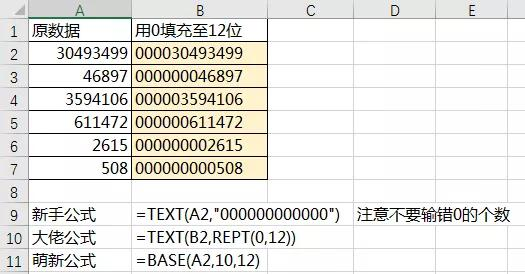(BASE2参数为指定的进制数，这个用法下数据10进制不变，故2参数固定为10(进制))。2，定位最后1个”-”的位置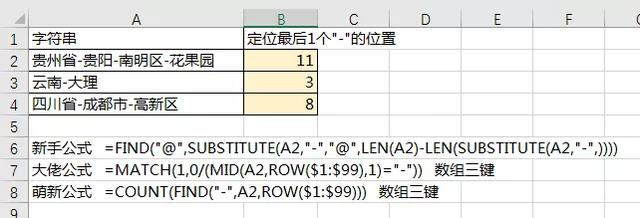3，日期类计算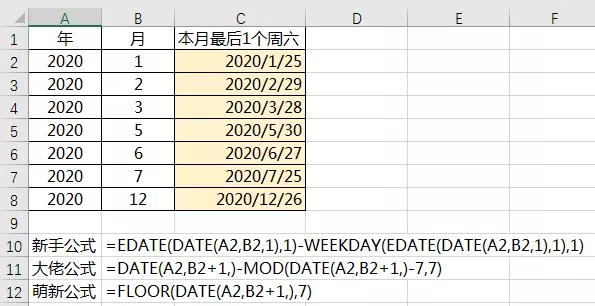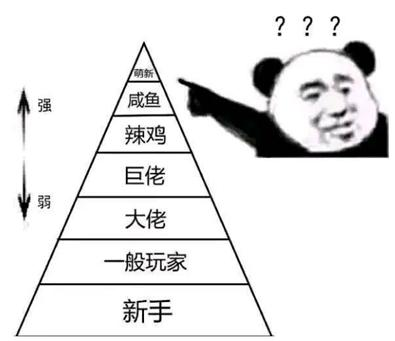4，日期类计算Ⅱ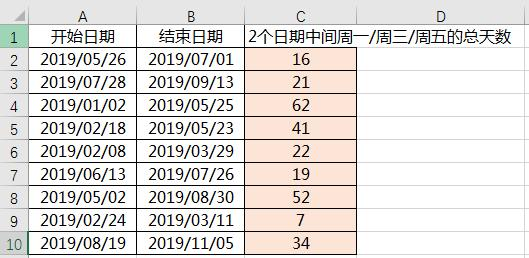=SUMPRODUCT(N(WEEKDAY(ROW(INDIRECT(A2&”:”&B2)),2)=1))

+SUMPRODUCT(N(WEEKDAY(ROW(INDIRECT(A2&”:”&B2)),2)=3))

+SUMPRODUCT(N(WEEKDAY(ROW(INDIRECT(A2&”:”&B2)),2)=5))

=SUMPRODUCT(N(WEEKDAY(ROW(INDIRECT(A2&”:”&B2)),2)={1,3,5}))

=NETWORKDAYS.INTL(A2,B2,”0101011″)”萌新”总是能找到正确的函数做正确的事情，利用NETWORKDAYS.INTL的3参数利用1和0的7位数序列来指定控制计算周几。

(周末字符串值的长度为七个字符，并且字符串中的每个字符表示一周中的一天(从星期一开始)｡ 1 表示非工作日，0 表示工作日｡ 在字符串中仅允许使用字符 1 和 0｡ 使用 1111111 将始终返回0｡

5，判断胜负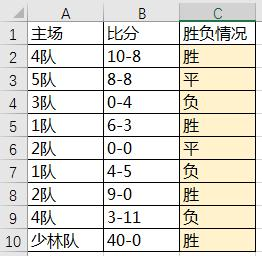=IF(–LEFT(B2,FIND(“-“,B2)-1)>–MID(B2,FIND(“-“,B2)+1,9),”胜”,IF(–LEFT(B2,FIND(“-“,B2)-1)=–MID(B2,FIND(“-“,B2)+1,9),”平”,”负”))

=TEXT(LEFT(B2,FIND(“-“,B2)-1)-MID(B2,FIND(“-“,B2)+1,9),”胜;负;平”)

=TEXT(IMREAL(IMDIV(B2&”I”,”1+I”)),”胜;负;平”)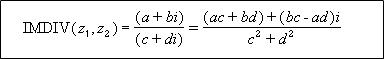(利用对应参数C+DI实现AC+BD 为A-B算法，用IMREAL提取复数结构中的实部系数，即为需要的2个数的差值的正负情况。6，最大值之和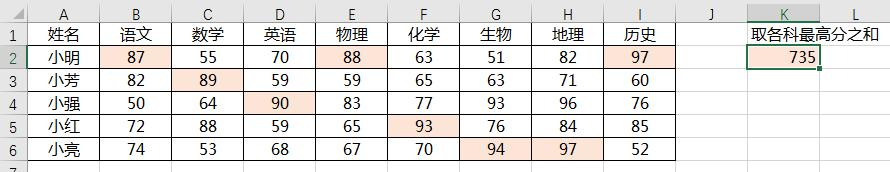=MAX(B2:B6)+MAX(C2:C6)+MAX(D2:D6)+MAX(E2:E6)+MAX(F2:F6)+MAX(G2:G6)+MAX(H2:H6)+MAX(I2:I6)

=SUM(SUBTOTAL(4,OFFSET(A2:A6,,COLUMN(A:H))))

(注意数组三键)

=SUM(DMAX(A1:I6,COLUMN(B:I),Z1:Z2))

(注意数组三键，Z1:Z2是任意空白区域，利用数据库函数性质默认条件为全部)

7，不规范时间格式处理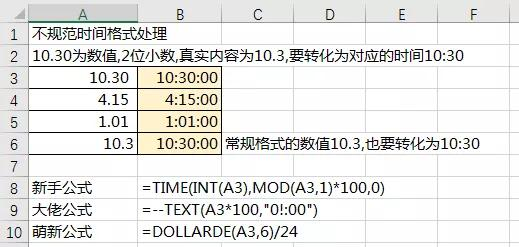“萌新”利用财务函数DOLLARDE对小数部分进行进制换算后直接用时间计算方式(1天是24小时)处理成正确时间。